# Functions for Coated Spheres (Core-Shell Particles)¶

MieQCoreShell(mCore, mShell, wavelength, dCore, dShell[, nMedium=1.0, asDict=False, asCrossSection=False])

Compute Mie efficencies Q and asymmetry parameter g of a single, coated particle. Uses CoreShell_ab() to calculate an and bn , and then calculates Qi following closely from the original BHMIE.

The code will return a warning if a non-unity nMedium is specified, since it was added naively. The mathematics to compute the effective index is the same as in the homogeneous particle functions.

Parameters

mCore : complex
The complex refractive index of the core region, with the convention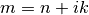.
mShell : complex
The complex refractive index of the shell region, with the convention.
wavelength : float
The wavelength of incident light, in nanometers.
dCore : float
The diameter of the core, in nanometers.
dShell : float
The diameter of the shell, in nanomaters. This is equal to the total diameter of the particle.
nMedium : float, optional
The refractive index of the medium. This must be positive, nonzero, and real. Any imaginary part will be discarded.
asDict : bool, optional
If True, returns the results as a dict.
asCrossSection : bool, optional
If specified and set to True, returns the results as optical cross-sections with units of nm2.

Returns

qext, qsca, qabs, g, qpr, qback, qratio : float
The Mie efficencies described above.
cext, csca, cabs, g, cpr, cback, cratio : float
If asCrossSection==True, MieQCoreShell() returns optical cross-sections.
q : dict
If asDict==True, MieQCoreShell() returns a dict of the above efficiencies with appropriate keys.
c : dict
If asDict==True and asCrossSection==True, returns a dict of the above cross-sections with appropriate keys.

Considerations

When using this function in a script, there are three simplifying clauses that can speed up computation when considering both coated and homogeneous particles. Upon determining the size parameters of the core and the shell:

CoreShellScatteringFunction(mCore, mShell, wavelength, dCore, dShell[, nMedium=1.0, minAngle=0, maxAngle=180, angularResolution=0.5, normed=False])

Computes the angle-dependent scattering intensity of a coated sphere.

Parameters

mCore : complex
The complex refractive index of the core region, with the convention.
mShell : complex
The complex refractive index of the shell region, with the convention.
wavelength : float
The wavelength of incident light, in nanometers.
dCore : float
The diameter of the core, in nanometers.
dShell : float
The diameter of the shell, in nanomaters. This is equal to the total diameter of the particle.
nMedium : float, optional
The refractive index of the medium. This must be positive, nonzero, and real. Any imaginary part will be discarded.
minAngle : int, optional
The minimum scattering angle returned by the calculations.
maxAngle : int, optional
The maximum scattering angle returned by the calculations.
angularResolution : float, optional
The angular resolution returned by the calculations.
normed : bool, optional
Set to True to return normalized results.

Returns

theta : numpy.ndarray
An array of the angles used in calculations. Values will be spaced according to angularResolution, and the size of the array will be (maxAngle-minAngle)/angularResolution.
SL : numpy.ndarray
An array of the scattered intensity of left-polarized (parallel) light. Same size as the theta array.
SR : numpy.ndarray
An array of the scattered intensity of right-polarized (perpendicular) light. Same size as the theta array.
SU : numpy.ndarray
An array of the scattered intensity of unpolarized light, which is the average of SL and SR. Same size as the theta array.
CoreShellS1S2(mCore, mShell, xCore, xShell, mu)

Computes S1 and S2 of a coated sphere as a function of mu, the cosine of the scattering angle.

Parameters

mCore : complex
The complex refractive index of the core region, with the convention.
mShell : complex
The complex refractive index of the shell region, with the convention.
wavelength : float
The wavelength of incident light, in nanometers.
dCore : float
The diameter of the core, in nanometers.
dShell : float
The diameter of the shell, in nanomaters. This is equal to the total diameter of the particle.
mu : float
The cosine of the scattering angle.

Returns

S1, S2 : complex
The S1 and S2 values.
CoreShellMatrixElements(mCore, mShell, xCore, xShell, mu)

Calculates the four nonzero scattering matrix elements S11, S12, S33, and S34 as functions of μ=cos(θ), where θ is the scattering angle: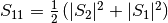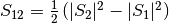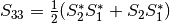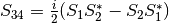Parameters

mCore : complex
The complex refractive index of the core region, with the convention.
mShell : complex
The complex refractive index of the shell region, with the convention.
wavelength : float
The wavelength of incident light, in nanometers.
dCore : float
The diameter of the core, in nanometers.
dShell : float
The diameter of the shell, in nanomaters. This is equal to the total diameter of the particle.
mu : float
The cosine of the scattering angle.

Returns

S11, S12, S33, S34 : float
The matrix elements described above.